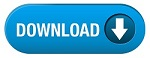Home » SSC » Model Questions for Scientific Assistant (IMD) Exam (Paper-2) PHYSICS – Set 39

# Model Questions for Scientific Assistant (IMD) Exam (Paper-2) PHYSICS – Set 39## 1. The tangential component of an electric field will be continuous in which boundary?

a) Conductor-Conductor
b) Conductor-Dielectric
c) Dielectric-Dielectric
d) Any boundary

a) Conductivity
b) Permeability
c) Wavelength
d) Permittivity

## 3. Magnetic vector potential is a vector—-

a) Whose curl is equal to the magnetic flux density
b) Whose curl is equal to the electric field intensity
c) Whose divergence is equal to electric potential
d) Which is equal to the vector product E x H

a) Y direction
b) Z direction
c) X direction
d) XY direction

## 5. An electric field on a plane is described by its potential V = 20(r-1 + r-2), where r is the distance from the source. The field is due to—-

a) A monopole
b) A dipole
c) Both a monopole and a dipole

### 6. The electric field strength at a far-off point P due to a point charge, +q located at the origin O is 100 milliVolt/meter. The point charge is now enclosed by a perfectly conducting hollow metal sphere with its centre and the origin O. The electric field strength at the point, P is—-

a) Remains unchanged in its magnitude and direction
b) Remains unchanged in its magnitude but reverse in direction
c) Would be that due to a dipole formed by the charge, +q, at O and -q induced
d) Would be zero

## 7. The electric field of a uniform plane electromagnetic wave in free space, along the positive direction, is given by E = 10(ay + jaz)e-j25x. The frequency and polarization of the wave, respectively are—-

a) 1.2 GHz, left circular
b) 4 GHz, left circular
c) 1.2 GHz, right circular
d) 4 GHz, right circular

## 8. Identify which one of the following will NOT satisfy the wave equation?

a) 50ej(wt – 3z)
b) sin(w(10z + 5t))
c) cos(y2 + 5t)
d) sin x cos t

## 9. The intrinsic impedance of copper at high frequencies is—-

a) Purely resistive
b) Purely inductive
c) Complex with a capacitive component
d) Complex with a inductive component

## 10. A loop is rotating about the y axis in a magnetic field B = Bocos(wt +a)at. The voltage in the loop is—-

a) Zero
b) Due to rotation only
c) Due to transformer action only
d) Due to both rotation and transformer action

#